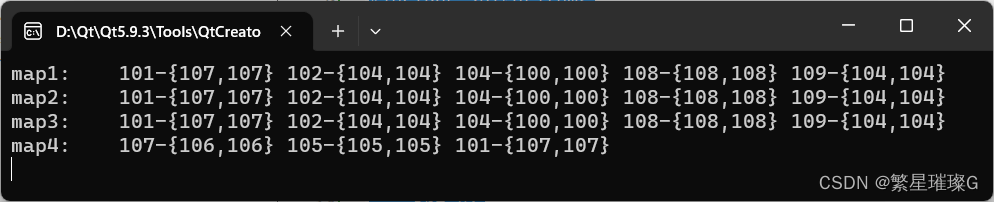# c++11 standard template (STL) std::map (3)

 template< class Key, class T, class Compare = std::less, class Allocator = std::allocator > > class map; (1) namespace pmr { template > using map = std::map std::pmr::polymorphic_allocator>> } (2) (since C++ 17)

`std::map` is an ordered key-value pair container, the key of its elements is unique. Sort keys with comparison function `Compare`. Search, remove, and insert operations have logarithmic complexity. Maps are usually implemented as red-black trees.

In every place where the standard library uses the Compare concept, uniqueness is checked with an equivalence relation. Imprecisely, if two objects `a` and `b` are not smaller than each other when compared: `!comp(a, b) & amp; & amp; !comp (b, a)` , they are considered equivalent (not unique).

`std::map` satisfies Container (Container), AllocatorAwareContainer (AllocatorAwareContainer), AssociativeContainer (AssociativeContainer ) and Reversible Container (ReversibleContainer) requirements.

## Assign to container

`std::map<Key,T,Compare,Allocator>::operator=`
 map & amp; operator=( const map & amp; other ); (1) map & amp; operator=( map & amp; & amp; other ); (2) (since C++11) (until C++17) map & amp; operator=( map & amp; & amp; other ) noexcept(/* see below */); (since C++17) map & operator=( std::initializer_list ilist ); (3) (since C++ 11)

Replace container contents.

1) Copy assignment operator. Replace the content with a copy of `other`. If std::allocator_traits::propagate_on_container_copy_assignment::value is true , replaces the target allocator with a copy of the source allocator. If the source and target allocators do not compare equal, the memory is destroyed with the target ( *this ) allocator, then allocated with `other`‘s allocator before copying the element. (since C++11).,

2) Move assignment operator. Replace the content with the content of `other` using move semantics (i.e. move the data in `other` from `other` to this container). After `other` is in a legal but unspecified state. If std::allocator_traits::propagate_on_container_move_assignment::value is true , replaces the target allocator with a copy of the source allocator. If it is false and the source and target allocators do not compare equal, the target cannot take ownership of the source memory, but must move assignments element-by-element individually, allocating additional memory as needed with its own allocator. In any case, the element originally in *this is either destroyed or replaced with an element-wise move assignment.

3) Replace the contents with those identified by initializer_list `ilist`.

### parameter

 other – Another container to use as a data source ilist – initializer_list used as data source

*this

### Complexity

1) Linear in size of `*this` and `other`.

2) Linear in the size of `*this`, unless the allocator does not compare equal and does not propagate, in which case it is the same as that of `*this` and `other` The size is linear.

3) Typically O(NlogN) where N is size() + ilist.size() . Linear if ilist is sorted with respect to value_comp().

### Exception

2) noexcept rules:

noexcept(std::allocator_traits::is_always_equal::value
& amp; & amp; std::is_nothrow_move_assignable::value)

(since C++17)

## Returns the associated allocator

`std::map<Key,T,Compare,Allocator>::get_allocator`
 allocator_type get_allocator() const;

Returns the allocator associated with the container.

(none)

### Return value

The associated allocator.

constant.

## Call example

```#include <iostream>
#include <forward_list>
#include <string>
#include <iterator>
#include <algorithm>
#include <functional>
#include <map>
#include <time.h>

using namespace std;

struct Cell
{
int x;
int y;

Cell() = default;
Cell(int a, int b): x(a), y(b) {}

Cell & amp; operator + =(const Cell & amp; cell)
{
x + = cell.x;
y + = cell.y;
return *this;
}

Cell & amp; operator + (const Cell & amp; cell)
{
x + = cell.x;
y + = cell.y;
return *this;
}

Cell & amp; operator *(const Cell & amp; cell)
{
x *= cell.x;
y *= cell.y;
return *this;
}

Cell & operator + + ()
{
x + = 1;
y + = 1;
return *this;
}

bool operator <(const Cell & cell) const
{
if (x == cell.x)
{
return y < cell.y;
}
else
{
return x < cell.x;
}
}

bool operator >(const Cell & cell) const
{
if (x == cell.x)
{
return y > cell.y;
}
else
{
return x > cell.x;
}
}

bool operator ==(const Cell & cell) const
{
return x == cell.x & amp; & amp; y == cell.y;
}
};

struct myCompare
{
bool operator()(const int & amp; a, const int & amp; b)
{
return a < b;
}
};

std::ostream & amp;operator<<(std::ostream & amp;os, const Cell & amp;cell)
{
os << "{" << cell.x << "," << cell.y << "}";
return os;
}

std::ostream & amp;operator<<(std::ostream & amp;os, const std::pair<const int, Cell> & amp;pCell)
{
os << pCell.first << "-" << pCell.second;
return os;
}

int main()
{
auto genKey = []()
{
return std::rand() % 10 + 100;
};

auto generate = []()
{
int n = std::rand() % 10 + 100;
Cell cell{n, n};
return cell;
};

std::map<int, Cell> map1;
for (size_t index = 0; index < 5; index ++ )
{
map1.insert({genKey(), generate()});
}
std::cout << "map1: ";
std::copy(map1.begin(), map1.end(),
std::ostream_iterator<std::pair<const int, Cell>>(std::cout, " "));
std::cout << std::endl;

//1) Copy assignment operator. Replace the content with a copy of other.
std::map<int, Cell> map2 = map1;
std::cout << "map2: ";
std::copy(map2.begin(), map2.end(),
std::ostream_iterator<std::pair<const int, Cell>>(std::cout, " "));
std::cout << std::endl;

//2) Move assignment operator. replaces the content with the content of other using move semantics
std::map<int, Cell> map3 = std::move(map1);
std::cout << "map3: ";
std::copy(map3.begin(), map3.end(),
std::ostream_iterator<std::pair<const int, Cell>>(std::cout, " "));
std::cout << std::endl;

//3) Replace the contents with those identified by the initializer_list ilist.
std::map<int, Cell, std::greater<int>> map4({<!-- -->{genKey(), generate()}, {genKey(), generate()},
{genKey(), generate()}, {genKey(), generate()}, {genKey(), generate()}});
std::cout << "map4: ";
std::copy(map4.begin(), map4.end(),
std::ostream_iterator<std::pair<const int, Cell>>(std::cout, " "));
std::cout << std::endl;
return 0;
}```

### Output